# Find An Equation For A Tangent Line (3 Key Steps)

In calculus, the slope of a tangent line tells us how steep the curve is at a given point.  We can use this information to find out how quickly something is changing, or if we have a local maximum or minimum.

So, how do you find an equation for a tangent line?  To find an equation for a tangent line, first find the derivative function f’(x) for the function f(x). Next, find the slope of the tangent line at the point (c, d), which is f’(a). Last, plug in the values m = f’(c), x = c, and y = d in the linear equation y = mx + b to solve for the y-intercept b.

Of course, you will need to know the various rules for taking derivatives (power rule, etc.) to find derivative functions and tangent lines.

In this article, we’ll talk about how to find the equation for a tangent line, step by step.  We’ll also look at various examples so you can see how it works.

Let’s get started.

## Find An Equation For A Tangent Line

To find the equation of a tangent line for a function f(x) at the point (c, d), there are three basic steps to follow:

• 1. Take the derivative of the function f(x).  This will give us the derivative function f’(x).
• 2. Substitute x = c into the derivative function to get f’(c), which is the slope of the tangent line.
• 3. Substitute m = f’(c), x = c, and y = d into the equation y = mx + b, and then solve for b, which is the y-intercept of the tangent line.

Remember that the tangent line has the form y = mx + b (since it is a line).  So, all we need to do is find numerical values for m (the slope) and b (the y-intercept) to specify a tangent line.

### Example 1: Find An Equation For A Tangent Line To A Parabola (Quadratic)

Consider the quadratic function f(x) = 3x2 – 5x + 8 (whose graph is a parabola).  What is the equation of the tangent line to the curve at the point (2, 10)?

Let’s follow the steps listed above to find the tangent line.

First, we take the derivative of f(x) = 3x2 – 5x + 8.  Using the power rule for each term, we get:

• f’(x) = 6x – 5 + 0
• f’(x) = 6x – 5

Next, we substitute x = 2 into the derivative function f’(x) to get:

• f’(x) = 6x – 5
• f’(2) = 6(2) – 5 = 12 – 5 = 7

Finally, we substitute m = f’(2) = 7, x = 2, and y = 10 into the equation y = mx + b and solve for b to get:

• y = mx + b
• 10 = 7(2) + b
• 10 = 14 + b
• -4 = b

Now, we use m = f’(2) = 7 and b = -4 in our tangent line equation to get:

• y = mx + b
• y = 7x – 4

So, the equation of the tangent line to the curve f(x) = 3x2 – 5x + 8  at the point (2, 10) is y = 7x – 4.

You can see this illustrated on the graph below.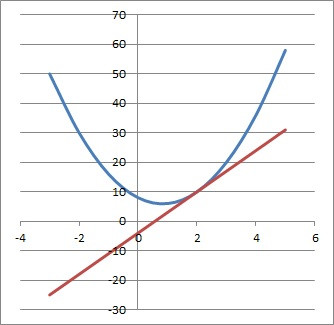Here we see the graph of the quadratic function f(x) = 3x2 – 5x + 8 (blue curve) and the tangent line y = 7x – 4 (red line) at the point (2, 10).

### Example 2: Find An Equation For A Tangent Line To A Cubic

Consider the cubic function f(x) = 4x3 – 5x2 + 2x – 9.  What is the equation of the tangent line to the curve at the point (1, -8)?

Let’s follow the steps listed above to find the tangent line.

First, we take the derivative of f(x) = 4x3 – 5x2 + 2x – 9.  Using the power rule for each term, we get:

• f’(x) = 12x2 – 10x + 2 – 0
• f’(x) = 12x2 – 10x + 2

Next, we substitute x = 1 into the derivative function f’(x) to get:

• f’(x) = 12x2 – 10x + 2
• f’(1) = 12(1)2 – 10(1) + 2
• f’(1) = 12 – 10 + 2
• f’(1) = 4

Finally, we substitute m = f’(1) = 4, x = 1, and y = -8 into the equation y = mx + b and solve for b to get:

• y = mx + b
• -8 = 4(1) + b
• -8 = 4 + b
• -12 = b

Now, we use m = f’(1) = 4 and b = -12 in our tangent line equation to get:

• y = mx + b
• y = 4x – 12

So, the equation of the tangent line to the curve f(x) = 4x3 – 5x2 + 2x – 9  at the point (1, -8) is y = 4x – 12.

You can see this illustrated on the graph below.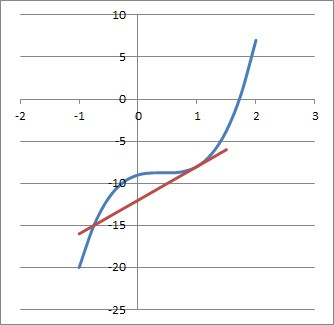Here we see the graph of the cubic function f(x) = 4x3 – 5x2 + 2x – 9 (blue curve) and the tangent line y = 4x – 12 (red line) at the point (1, -8).

### Example 3: Find An Equation For A Tangent Line To An Exponential Function

Consider the exponential function f(x) = 2x.  What is the equation of the tangent line to the curve at the point (4, 16)?

Let’s follow the steps listed above to find the tangent line.

First, we take the derivative of f(x) = 2x.  Using the rule for exponential functions, we get:

• f’(x) = ln(2)*2x

Next, we substitute x = 4 into the derivative function f’(x) to get:

• f’(x) = ln(2)*2x
• f’(4) = ln(2)*24
• f’(4) = ln(2)*16
• f’(4) = 16ln(2)

Finally, we substitute m = f’(4) = 16ln(2), x = 4, and y = 16 into the equation y = mx + b and solve for b to get:

• y = mx + b
• 16 = 16ln(2)(4) + b
• 16 = 64ln(2) + b
• 16 – 64ln(2) = b

Now, we use m = f’(4) = 16ln(2) and b = 16 – 64ln(2) in our tangent line equation to get:

• y = mx + b
• y = (16ln(2))x + (16 – 64ln(2))

So, the equation of the tangent line to the curve f(x) = 2x at the point (4, 16) is y = 16ln(2)x + 16 – 64ln(2).

You can see this illustrated on the graph below.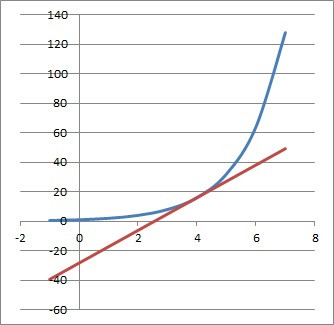Here we see the graph of the cubic function f(x) = 2x (blue curve) and the tangent line y = 16ln(2)x + 16 – 64ln(2) (red line) at the point (4, 16).

### Example 4: Find An Equation For A Tangent Line To A Trigonometric Function

Consider the trigonometric function f(x) = 4sin(x).  What is the equation of the tangent line to the curve at the point (π/6, 2)?

Let’s follow the steps listed above to find the tangent line.

First, we take the derivative of f(x) = 4sin(x).  Using the rule for sine functions, we get:

• f’(x) = 4cos(x)

Next, we substitute x = π/6 into the derivative function f’(x) to get:

• f’(x) = 4cos(x)
• f’(π/6) = 4cos(π/6)
• f’(π/6) = 4(√3/2)
• f’(π/6) = 2√3

Finally, we substitute m = f’(π/6) = 2√3, x = π/6, and y = 2 into the equation y = mx + b and solve for b to get:

• y = mx + b
• 2 = (2√3)(π/6) + b
• 2 = (2π√3/6) + b
• 1 = π√3/6 + b
• 1 – (π√3/6) = b

Now, we use m = f’(π/6) = 2√3 and b = 1 – (π√3/6) in our tangent line equation to get:

• y = mx + b
• y = 2√3x + 1 – (π√3/6)

So, the equation of the tangent line to the curve f(x) = 4sin(x) at the point (π/6, 2) is y = 2√3x + 1 – (π√3/6).

You can see this illustrated on the graph below.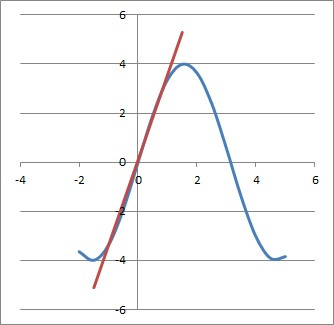Here we see the graph of the trigonometric function f(x) = 4sin(x) (blue curve) and the tangent line y = 2√3x + 1 – (π√3/6) (red line) at the point (π/6, 2).

### Example 5: Find An Equation For A Tangent Line To A Circle

Consider the circle given by the equation x2 + y2 = 16. What is the equation of the tangent line to the circle at the point (2√3, 2)?

Let’s follow the steps listed above to find the tangent line.

First, we take the derivative of x2 + y2 = 1 with respect to x.  This will require the use of implicit differentiation, using the notation y’ in place of f’(x):

• x2 + y2 = 1
• 2x + 2yy’ = 0
• 2yy’ = -2x
• y’ = -x/y

Next, we substitute x = 2√3 and y = 2 into the derivative function y’ to get:

• y’ = -x/y
• y’ = -(2√3)/2
• y’ = -√3

Finally, we substitute m = y’(2√3, 2) = -√3, x = 2√3, and y = 2 into the equation y = mx + b and solve for b to get:

• y = mx + b
• 2 = (-√3)(2√3) + b
• 2 = -6 + b
• 8 = b

Now, we use m = y’(2√3, 2) = -√3 and b = 8 in our tangent line equation to get:

• y = mx + b
• y = (-√3)x + 8

So, the equation of the tangent line to the circle x2 + y2 = 16 at the point (2√3, 2) is y = (-√3)x + 8.

You can see this illustrated on the graph below.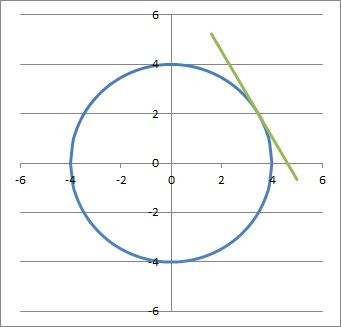Here we see the graph of the circle x2 + y2 = 16 (blue curve) and the tangent line y = (-√3)x + 8 (green line) at the point (2√3, 2).

## Conclusion

Now you know how to find the equation of a tangent line for a given function (or curve).  You also know about some special cases to look out for.

You can learn about how to use derivatives and graphs to find function maximums here.